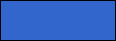Spin Electronics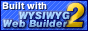Magnetic Materials at Nanoscale
Optical Spectroscopies
Simulations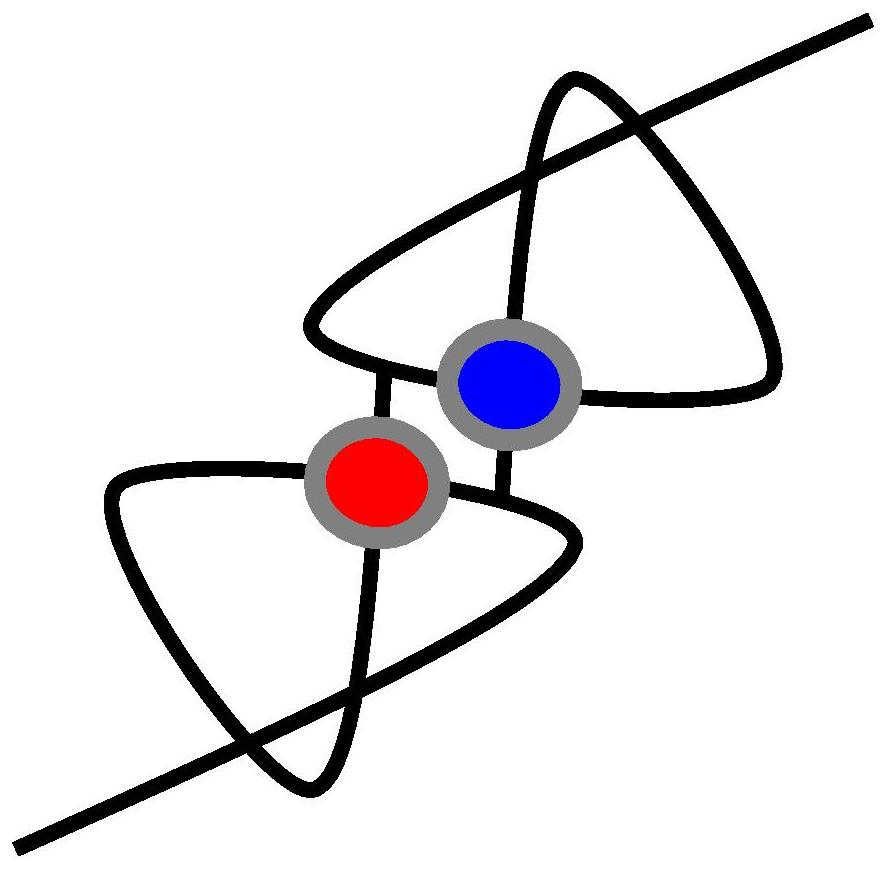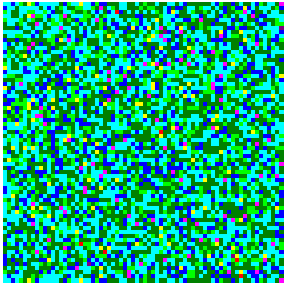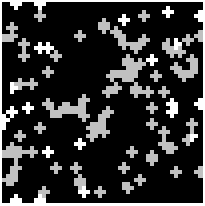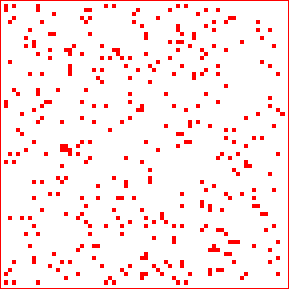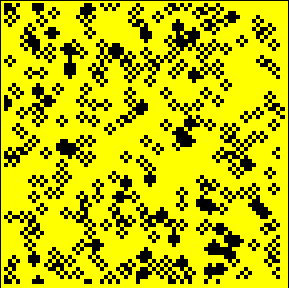The simulation of exchange bias at the ferromagnetic/antiferromagnetic interface - the Random Field Ising Model
In the figures the randomly distributed atomic roughness at the FM/AFM interface is seen. In the right the configuration of spins after one cycle of hysteresis loop creation is provided. There are two types of magnetic clusters: those which follows the exchange bias (grey squares) and those which were biased in the opposite direction (white squares).
In the figures the randomly distributed places of increased exchange interaction between FM spins are seen (on the left). In the right figure the Heisenberg energy distribution being in the state influenced by the maximized magnetic field, applied in the oppoiste to initial saturation state, is visible. The black squares mark the +1 spins, while the -1 squaress are yellow.
The RFIM simulator ver.02 (70x70 lattice),  The RFIM simulator ver.03a (100x100 lattice), The RFIM simulator ver.03b (100x100 lattice, no Gauss field),
The RFIM simulator ver. 02 (300x300 lattice) black&white - it shows spins unreversed from the initial saturated state (grey) and the spins not reversed from the negative saturated state (white) - details can be found in T. Błachowicz, CEJP 4, 3 (2006), 331-340.
EL - this is a link for the Brillouin light scattering simulator - 4 layers + substrate sample (exe file).
SL - this is a link for the Brillouin light scattering simulator - a single layer + substrate sample (exe file).
The simulations of driven, dumped pendulum revealing the chaotic behavior (deterministic chaos)
Presented below phase maps were generated by the two simple simulators which design and functionality were inspired by the book Chaotic Dynamics: an introduction by G. L. Baker and J. P. Gollub, Cambridge University Press 1996. The simulators are very similar: the first one (the trajectory simulator) is useful for a phase trajectory observations in time (you can skip a given number of preliminary simulation steps at the initial state), while in the second simulator (the Poincare sections creator), which produces Poincare sections, you can adjust a pixel dimension what is especially suitable in a case of a simple Poincare sections.

Both programs a free for downloads, for didactic or research purposes only - in such a case, please acknowledge the program author properly. The current versions are 1.1a, and 1.2a. for the trajectory simulator, and for the Poincare sections creator, respectively.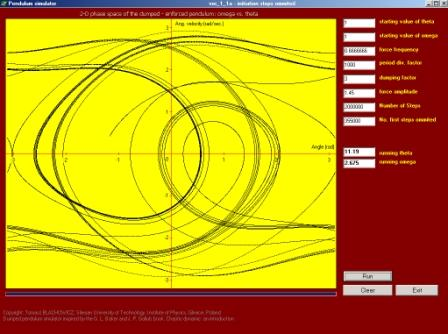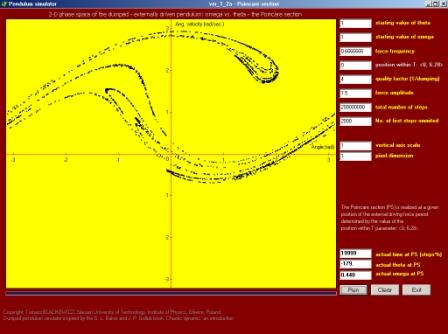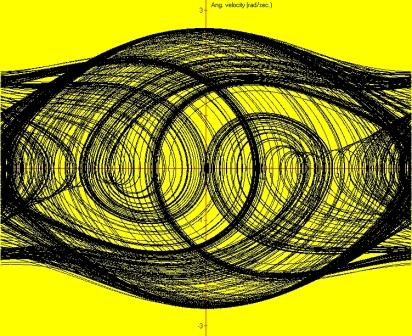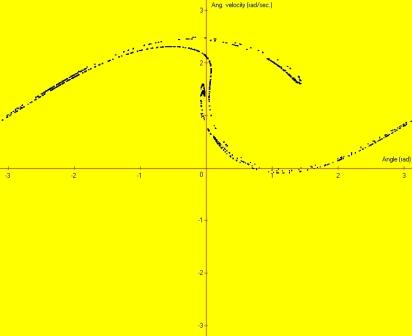The phase map of a pendulum: dumping=2, force=1.50
The Poincare section for the left case made at the force period =1